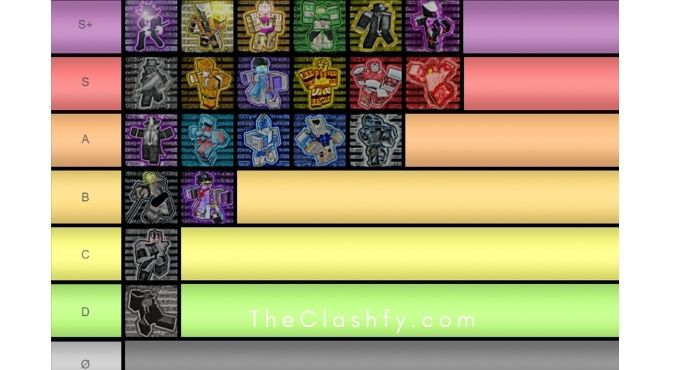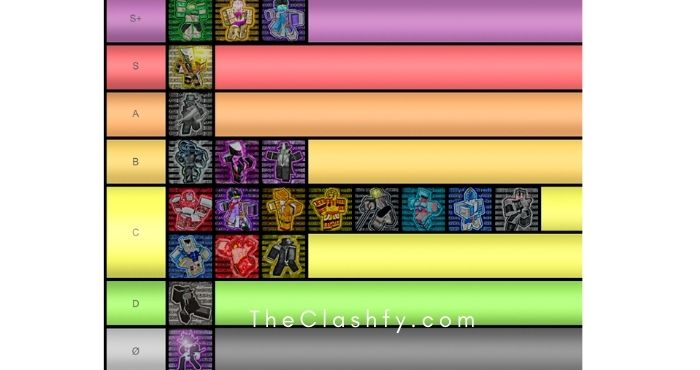Roblox

# In Another Time Trello & PvP Tier List 2022

Hi Guys, Welcome to our In Another Time Trello, Today, we will show you Tier List and Rarity Tier List for the game and Which is a Roblox game based on Jojo’s Bizarre adventure.

So come and take a look at this guide. Also, You can also check our Roblox Games Codes and Roblox Game Trello page.

Contents

## In Another Time Trello

### In Another Time PVP Tier List Wiki

This is the PVP tier list in overall 1v1s and wars, This is a general and rough estimation of stands that have more combos and damage output over other stands.### In Another Time Rarity Tier list Wiki

No one is obligated to follow the rarity tier list and everyone is free to trade for what they like.

##Combos Guide Wiki

Magician’s Red

• [ Y + R + T + H ]
10 + 25 + 20 + 25 = 80 damage

(This combo should be used/works better if your enemy is blocking)

• [ F + E + T + G + H ]
N/A + 80 + 20 + 20 + 25 = 145 damage

• [ R + T/G/H ]
25 + 20/20/25 = 45/50 damage

Sticky Fingers

• [ R + H + T + F + V ]
35 + 15 + 25 + 50 / 60 + 15 = 150 / 140 damage

(Use V if your enemy try to escape)

• [ R + T ]
35 + 25 = 60 damage

• [ F + T ]
50/60 + 25 = 75/85 damage

• [ V + E + R + T ]
15 + 80/120 + 35 + 25 = 155/195 damage

Silver Chariot

• [ R + F + E + T ]
35 + 20 + 60 + 25 = 140 damage

(This combo is very risky to pull off, because you can also get punished back since Silver Chariot lacks defense)

• [ Y + B ]
15 + 25 = 40 damage

(Remember to use the B move just in case your enemy gets closer to you, because the Y move leaves you defenseless for 5 seconds)

• [ R + E ]
35 + 60 = 95 damage

• [ F + R + E ]
20 + 35 + 60 = 115 damage

The World: Over Heaven

• [ Y + R ]
60 + 60 / 70 = 120 / 130 damage

(Use this combo to deal damage or to get closer to your enemy)

• [ Y + G ]
60 + 50 = 110 damage

(Use this combo to get closer to your enemy or if your enemy block is broken)
(You can also use the combo backwards, G + Y, because the G move will force the enemy to block and after that they won’t be able to block the Y move)

• [ R + V ]
60/70 + 30 = 90/100 damage

• [ T + R ]
67.5 + 60/70 = 127.5/137.5 damage

• [ G + Y + R ]
50 + 60 + 60/70 = 170/180 damage

— Vampire —
• [ R + G + F ]
30 + 32.5 + 85 = 147.5 damage

• [ F + T ]
85 + 20 = 105 damage

(Your enemy will get knockback from the F move and your chances to land the T move will increase)

• [ F + T + C + G + R ]
85 + 20 + N/A + 32.5 + 30 = 167.5 damage

• [ T + G + R ]
20 + 32.5 + 30 = 82.5 damage

• [ G + R ]
32.5 + 30 = 62.5 damage

The World

• [ R + V + H + B ]
40 + N/A + 45 + 40 = 125 damage

(Use V to get closer to your enemy and continue your combo)

• [ B + Y ]
40 + 60 = 100 damage

(This combo works the best if your enemy block is broken, but even without it, it still deals good damage)

• [ Y + G ]
60 + 50 = 110 damage

(Use this combo to get closer to your enemy or if your enemy block is broken)
(You can also use the combo backwards, G + Y, because the G move will force the enemy to block and after that they won’t be able to block the Y move)

• [ R + T ]
40 + 22.5 = 62.5 damage

• [ B + H + R + T ]
40 + 45 + 40 + 22.5 = 147.5 damage

• [ G + C + V + B + H + R ]
50 + N/A + N/A + 40 + 45 + 40 = 175 damage

(C and V play a important role in this combo and should be used to get closer to your enemy)

• [ H + R + V + B + G + Y ]
45 + 40 + N/A + 40 + 50 + 60 = 235 damage

Star Platinum

• [ T + R + G ]
50 + 50 + 72 = 172 damage

• [ Y + T + G ]
30 + 50 + 72 = 152 damage

(Even if the Y move is a projectile, is better to use it when you are close to your enemy)

• [ T + G ]
50 + 72 = 122 damage

— Crazy Diamond —
• [ T + G ]
20 + 20 = 40 damage

• [ B + R ]
40 + 35 = 75 damage

• [ V + G/R/H ]
15 + 20/35/45 = 35/50/60 damage

• [ H + T ]
45 + 20 = 65 damage

• [ F + G + F + H + E + T ]
N/A + 35 + N/A + 45 + 100 + 20 = 200 damage

The Hand

• [ T + H ]
55+ 65 = 120 damage

• [ Y + R + T + H ]
N/A + 35 + 55 + 65 = 155 damage

(Use this only if you have to pull your enemy closer)

• [ F + G + T + H ]
50 + 80 + 55 + 65 = 250 damage

Star Platinum: The World

• [ R + V + H ]
45 + N/A + 40 = 85 damage

Golden Experience

• [ G + C + R ]
50 + N/A + 30 = 80 damage

• [ T + G + C + R ]
35 + 50 + N/A + 30 = 115 damage

King Crimson

• [ E + R ]
45 + 40 = 85 damage

• [ R + T ]
40 + 5 = 45 damage

(Use this combo to be able to blind your enemy easier)

• [ V + E + Y + R ]
N/A + 45 + 70 + 40 = 155 damage

(Use V only if you aren’t close to your enemy)

• [ Y + LMB + T + C + E ]
60 + 49 + 5 + N/A + 50 = 164 damage

White Snake

• [ R + T ]
35 + 20 = 55 damage

• [ G + E ]
20 + 60 = 80 damage

• [ F + E + R + T ]
25 + 60 + 35 + 20 = 140 damage

Hamon

• [ G + H + R + T ]
15 + 70 + 70 + 35/55 = 190/205 damage

• [ G + Y + R + T ]
15 + 35 + 50 + 25/45 = 125/145 damage

• [ Q + T + R + T + Y ]
N/A + 55 + 50 + 25/45 + 35 = 120/140 damage

Kars

• [ G + T ]
32.5 + 20 = 52.5 damage

• [ F + T ]
60 + 20 = 80 damage

• [ Q + E + T + F + G + R ]
N/A + 80.5 + 20 + 60 + 32.5 + 35 = 233 damage

Chariot Requiem

• [ T + Z ]
35 + 15/100 = 50/135 damage

(It depends on how many times you can hit your enemy with you Z)

• [ R + T + Z ]
35 + 35 + 15/100 = 85/170 damage

• [ H + R + T + Z ]
25 + 35 + 35 + 15/100 = 105/195 damage

• [ F + R + E + H ]
N/A + 35 + 25 + 25 = 85 damage

(You get the most damage out of sleep by using this)

Dio’s The World

• [ Y + G ]
60 + 50 = 110 damage

(Use this combo to get closer to your enemy or if your enemy block is broken)
(You can also use the combo backwards, G + Y, because the G move will force the enemy to block and after that they won’t be able to block the Y move)

• [ V + R + Y ]
N/A + 60/70 + 60 = 120/130 damage

• [ Q + V + F ]
N/A + N/A + 90 = 90 damage

(Use this to be sure your F will land on your enemy most of the time)

Gold Experience Requiem

• [ R + M1 + T ]
40 + 45 + 45/25 = 130/110 damage

• [ R + G + T ]
40 + 40 + 45/25 = 125/105 damage

• [ R + G + M1 + T]
40 + 40 + 45 + 45/25 = 170/150 damage

• [ G + C + Y + R + M1 + T ]
40 + N/A + 20 + 40 + 45 + 45/25 = 190/170 damage

(Use roll only if your enemy is far away from you)

• [ F + T ]
N/A + 45/25 = 45/25 damage

(After this combo, you can use any other combo from the above)

C-Moon

• [ Y + F ]
45 + 30 = 75 damage

• [ B + E ]
30/35 + 200 = 230/235 damage

(Because of the B move, the enemy will be unable to block your barrage, but that doesn’t mean you have time to perform a full one.)

• [ B + T ]
30/35 + 30 = 60/65 damage

(Use this if your enemy is far away from you. If they end up being close, follow the combo above.)

• [ V + Y + F ]
N/A + 45 + 30 = 75 damage

(Since V gives you extra speed and jump force, you will be able to hit them while they are stuck in the air.)

• [ V + Y + B ]
N/A + 45 + 30/35 = 75/80 damage

• [ Z + V + Y + F]
N/A + 45 + 30/35 = 75/80 damage

Similar to V + Y + F, this can be used as a combo starter due to Z’s enormous range. Although the enemy can be flung away breaking the combo

That’s it for this guide.